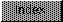```

NAME
dbf - convert FFP dual-binary number to FFP format

SYNOPSIS
fnum = dbf(exp, mant);

FUNCTION
Accepts a dual-binary format (described below) floating point
number and converts it to an FFP format floating point number.
The dual-binary format is defined as:

exp bit  16     = sign (0=>positive, 1=>negative)
exp bits 15-0   = binary integer representing the base
ten (10) exponent
man             = binary integer mantissa

INPUTS
exp - binary integer representing sign and exponent
mant - binary integer representing the mantissa

RESULT
fnum - converted FFP floating point format number

BUGS
None

```